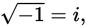# SAT Math Multiple Choice Question 401: Answer and Explanation

### Test Information

Question: 401

11. Given thatwhich of the following is equivalent to the sum i125 + i125?

• A. i14
• B. i250
• C. 2i45
• D. 2i250

Explanation:

C

Difficulty: Medium

Category: Additional Topics in Math / Imaginary Numbers

Strategic Advice: To evaluate a high power of i, look for patterns and use the definitionwhich, when written in a more useful form, is i2 = -1.

Getting to the Answer: Write out enough powers of i for you to see the pattern:Notice that the pattern (i, -1, -i, 1, i, -1, -i, 1) repeats on a cycle of 4. To evaluate i125, divide 125 by 4. The result is 31, remainder 1, which means 31 full cycles and then back to i1. This means i125 is equivalent to i1, which is i. Because i + i = 2i, you are looking for the answer choice that is also equivalent to 2i. Choices (C) and D look tempting (because of the 2), so start with them: (C) is correct because 45 ÷ 4 = 11, remainder 1, which means i45 is equivalent to i and 2i45 is equal to 2i.